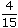# Aptitude - Ratio and Proportion - Discussion

Discussion Forum : Ratio and Proportion - General Questions (Q.No. 1)
1.
A and B together have Rs. 1210. Ifof A's amount is equal toof B's amount, how much amount does B have?
Rs. 460
Rs. 484
Rs. 550
Rs. 664
Explanation:

 4 A = 2 B 15 5A =2 x 15B 5 4A = 3 B 2A = 3 B 2A : B = 3 : 2.B's share = Rs.1210 x 2= Rs. 484. 5

Discussion:
143 comments Page 2 of 15.

Jyoti said:   1 year ago
Listen @Yogesh.

Take A=3x and B=2x.
5x= 1210 => x= 242.
Then B= 2 * 242= 484 Ans.
(12)

Akbar said:   1 year ago
Well done. Thanks for explaining.

Sindhu said:   1 year ago
Thank you @Ranjeet Kaur.

AKPARIDA said:   1 year ago
Cleared my doubt. Thank you, @Sridhar and @Bhuvi.

Utkalika said:   2 years ago
@Harshita

4/15 is on the left side.
So when we take it to the right side of the equal sign then we reciprocate it i.e., 4/15 becomes 15/4.

The same rule applies from right to left.

Harshita said:   2 years ago
Why 4/15 turned to 15/4? Please explain.

Yogesh said:   2 years ago
a:b = 3:2 then
b= 2/5 how? Explain.

Megha said:   2 years ago
As a total proportion of B is 2/5 of the total so the proportion of b is = 2.
and total proportion is 5; so A's proportion 5-2 = 3.
And B= 2/5 * total = 2/5*1210 = 484.
And A = 1210- 484 = 726.

Thanks.

Anomie said:   3 years ago
A+B = 1210.
(4/15)A = (2/5)B,
2A = 3B.
Therefore, A = (3B)/2,
and substituting, (3B/2) + B = 1210,
and hence, 5B=2420 => B = 484.

Mahender said:   3 years ago
Thanks @Sridhar.